# Standard Algorithm

Page 1 of 5
Let's just dive right in and do one!  I'm going to go really slowly and I'll show each step.  After you see a few examples, it's going to start making sense!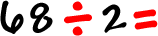The first thing we do is change the way the problem is written...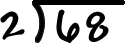The first math step is to look at that first number of the guy we are dividing into...  that 6This is the division step!

 We want to see how many times 2 will go into 6...  2 goes into 6 three times, right?  So, we put that 3 right above the 6:That wasn't hard at all, was it?

Here's the second step...  This is the multiplication step!

 Multiply the 3 and the 2 and put the answer right under the 6: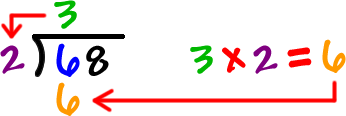Here's the third step...  This is the subtraction step!

 Do the subtraction...That's 6 - 6 = 0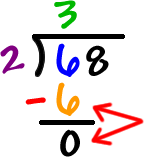Hey, we just finished the first chunk of steps!  And it wasn't that bad!

Division, then multiplication, then subtraction.
Let's call it the DMS loop!
Hey, those letters go alphabetically!!  You can use that to remember it!

OK, now we're going to do the exact same thing, but with a different number...

 First thing:  Drag the 8 down.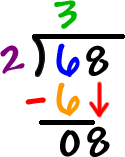Now, let's go back into our division, multiplication, subtraction loop using the 8!

 Division: We want to see how many times 2 will go into 8...  2 goes into 8 four times...  So, we put that 4 right above the 8: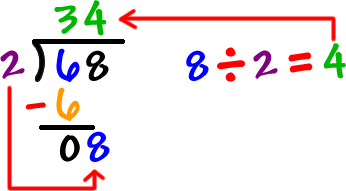Multiplication: Multiply the 4 and the 2 and put the answer right under the 8: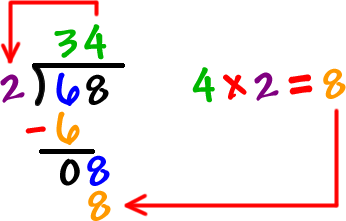Subtraction: That's 8 - 8 = 0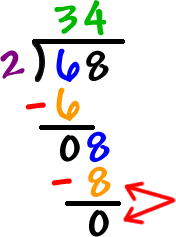Guess what?  We are DONE!  (No way.)  WAY!

 We used the 6 and the 8... and we ended with a 0 at the bottom... Which I made into a "happy face" because I was so happy to be done! The answer is 34.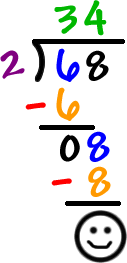So, we do our DMS loop (division-multiplication-subtraction) until we use all the numbers in the guy we are dividing into (that guy is officially called the dividend).

We have two ways to check whether our answer is right or not:

 1) Grab a calculator and do 68 divided by 2. 2) Use multiplication!  Remember that division and multiplication go together...  They undo each other!  So, 34 x 2 should = 68!  This is great practice for you.

Let's do a bunch more...  Go to the next page to do another one!